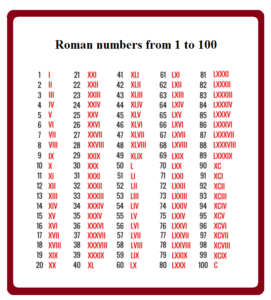September 30, 2023

# Roman Numbers

Contents

## What are Roman numbers?

The numerals 0, 1, 2, 3,…..,9 are used in writing are called Hindu -Arabic numerals. So far, we’ve used the Hindu-Arabic numeral system. This isn’t the only system available. The Roman numeral system is one of the first ways of writing numerals used by the Romans in the past. In many places, this system is still in use. For example, Roman numerals are used in clocks; they are also used for classes in school time tables,  used in numbering of different volumes of books , and so on.  Alphabets are used to write fixed positive integers in roman numbers.

There are seven basic symbols( I, V, X, L, C, D and  M) to write any Roman numbers. These symbols with their corresponding Hindu-Arabic numbers  are given below:

 Roman numeral I V X L C D M Hindu Arabic Numerals 1 5 10 50 100 500 1000

In the roman number system , there is no symbol for 0. This system is also not a place value system. Using these symbols, we can write any number by following certain rules which are given below:

### Rules  to write roman numbers.

Rule 1: (i) Repetition of a Roman  numbers  means addition.

(ii) Only  I, X , C and M can be repeated.

(iii) V, L and D cannot be repeated.

(iv) No Roman numeral can be repeated more than three times.

Examples: (i) XXX = 10+10+10= 30     (ii) CCC= 100+100+100=300

Rule 2:  A smaller numeral written to the right of a larger numeral is always added to the larger numeral.

Examples: (i) DX =500+10= 510             (ii) MCC = 1000+100+100=1200

Rule 3: (i) A smaller numeral written to the left of a larger numeral is always subtracted  from the larger numeral.

(ii)  The symbols V, L and D are never written to the left of a symbol of greater value, it  means V, L and D are never subtracted.

(iii) I can be subtracted from V and X only.

(iv) X can be subtracted from  L and C only.

(v) C can be subtracted  from  D and M only.

Examples: (i) XC=100-10=90      (ii)  CD=  500- 100 =400

Rule 4: When a smaller numeral is placed between two larger numerals, then it is always subtracted from the larger numeral immediately following it.

Examples: (i)  CXC = 100 +(100-10) =100+90=190     (ii) CXIV= 100+10+(5-1) =114

Rule 5: A bar placed over a number multiplies its value by 1,000.

Examples : (i)         (ii)

#### Roman numbers 1 to 100

Below is the chart for roman numbers from 1 to 100.##### Converting Roman Numerals to Hindu- Arabic numbers and vice-versa.

(a) Write the Roman numeral for each of the following Hindu-Arabic numerals:

(i) 189

Sol. 189= 100+80 +9 =  C+LXXX+IX = CLXXXIX

(ii)444

Sol.  444 = 400+40+4 =CD +XL+IV =CDXLIV

(iii) 169

Sol.  169=100+50+10+9= C+L+X+IX= CLXIX

(iv) 2359

Sol. 2359=2000+300+50+9 = MM+CCC+ L+IX = MMCCCLIX

(v) 18985

Sol. 18985 = 18000+900+80+5=    =                   (900 = 1000-100 =CM)

(b) Write the Hindu-Arabic numerals corresponding to each of the following:

(i)CCXLVII

Sol. CCXLVII  = CC +XL +VII = (100+100) +(50-10)+7 =200+40+7 = 247

(ii) CDXXXVIII

Sol. CDXXXVIII=  CD +XXX+VIII =(500-100) +(10+10+10) + 8 =400+ 30+8= 438

(iii) CCXCVI

Sol. CCXCVI = CC+XC +VI  =(100+100)+(100-10)+6 =200+90+6 =296

(iv) MMMCDVI

Sol. MMMCDVI =MMM+CD+VI =(1000+1000+1000)+(500-100) +6 =3000+400+6=3406

(v)

Sol.   = +CM+XX +I  = ( +(1000-100)+(10+10) +1 = 6000+900+20+1 =6921

###### Solved Examples on Roman Numbers

A. State whether each of the following statement is true or false.

(i) There is no symbol for zero in Roman number system.

(ii) Repetition of Roman  numbers means multiplication.

(iii) V , L and D can neither  be repeated nor subtracted.

(iv)No numeral can be repeated more than three times.

(v) I can be subtracted from C.

(vi)X can be subtracted from L.

(vii)C can be subtracted from M.

Ans. (i) True  (ii) False   (iii) True   (iv) True    (v) False   (vi)True  (vii) True

B. Why the following roman numerals are meaningless?

(i) VX

Sol. Since V is never written to  the left of a symbol of greater value.

(ii) VVIII

Sol. Since  V, L and D cannot be repeated.

(iii) IVC

Sol.  Since V is never written to  the left of a symbol of greater value.

(iv) LIL

Sol. Since  I can be subtracted from V and X only.

C. Solve and write the answers in Roman numerals

(i) XCII-XV

Sol. XCII= (100-10) +2 =92   and  XV=10+5=15

So XCII-XV = 92-15 =77 =  70+7= LXXVII

(ii) CVIII+CXLVI

Sol. CVIII= 100+8=108   and CXLVI= C+XL+VI= 100+(50-10)+6=100+40+6=146

Hence CVIII+CXLVI= 108+146= 254= 200+50+4= CCLIV

###### Frequently Asked Questions on Roman Numbers

(i) What number is XXL?

Sol.  XXL is not a valid numeral.

Proper Roman numerals proceed in descending order unless it’s a “subtractive” numeral.  A larger numeral should be written before a Subtractive numerals. A numeral of lower value, X ( = 10), may not stand in front of a group of numerals in subtractive notation, XL ( = 40).

(ii)What does MMXX stand for?

Sol. MMXX =1000+1000+10+10= 2020 . So MMXX stands for 2020.

(iii) What is the Roman number of 900?

Sol.  900 = 1000-100= CM . So in Roman numerals 900 is written as CM

(iv) How do you write 5000 in Roman numerals?

Sol. A bar placed over a number multiplies its value by 1,000. So 5000=5 x 1000= .

Hence 5000=

(v) What is the Roman number of 9000?

Sol.  9000= 9 x 1000= I̅X̅   ( A bar placed over a number multiplies its value by 1,000.)

9000 in roman numerals is I̅X̅.

You might also be interested in:#### Bina singh

View all posts by Bina singh →
ssc chsl 2023 tier 1 cut off NIRF Rankings 2023 : Top 10 Engineering colleges in India CBSE Compartment Exam 2023 Application Form SSC CGL 2023 Notification: Important Dates UPSC Recruitment 2023